# WBJEE 2016 Physics Previous Year Paper with Solutions

WBJEE (West Bengal Joint Entrance Examination) is an entrance examination held for students willing to join professional courses like B.Tech and B.Pharm in the state of West Bengal. On this page, we have provided the WBJEE 2016 Physics question paper with solutions. The questions are solved in a simple and easy to understand method by our experts. The PDF form of the solutions is also available here for download. Practising previous years’ question papers will help the candidates get familiar with the pattern of the question paper and gain confidence in solving the problems.

### WBJEE 2016 - Physics

Question 1: The current flowing through the 3 Ω resistor in the circuit is,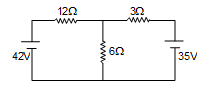1. a. 4.2A
2. b.3.0A
3. c. 3.5 A
4. d. 1.6A

Solution:

Applying Kvl in loop (1)

12i+6(i-i1)=42

In loop (2)

3i1-6(i- i1) =-35

From loop (1) equation and (2) equation.

⇒ 18i - 6i1 = 42 ...(3)

-6i + 9i1 = -35x3 ...(4)

Solving (3) and (4) we get,

⇒ 21 i1 = -63

⇒ i1 = -3

Question 2: A body attached to the lower end of a vertical spring oscillates with time period of 1 sec. The time period when two such springs are connected one below another is approximately

1. a. 0.7 sec
2. b. 1 sec
3. c. 1.4 sec
4. d. 2 sec

Solution:

Time period of oscillation is given by

$$T=2\pi \sqrt{\frac{m}{K}}$$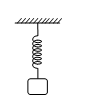Where

m = mass

K = spring constant

First case:-

So according to question

$$T=2\pi \sqrt{\frac{m}{K}}$$

Second case:-

When two springs are connected one below another then they will be in series therefore

$$\frac{1}{K_{eq}}=\frac{1}{K}+\frac{1}{K}$$

$$\frac{1}{K_{eq}}=\frac{K}{2}$$

$$T'= 2\pi \sqrt{\frac{m}{K_{eq}}}$$

$$K_{eq}=\frac{K}{2}$$

$$T'=\sqrt{2}T=1.414T$$

Question 3: The minimum and maximum capacitances, which may be obtained by the combination of three capacitors each of capacitance 6 μF are

1. a. 6 μF and 18 μF
2. b. 2 μF and 18 μF
3. c. 2 μF and 12 μF
4. d. 6 μF and 12 μF

Solution:

The minimum capacitance can be obtained by connecting all capacitor in series. It can be calculated as follows:

$$\frac{1}{C}=\frac{1}{6}+\frac{1}{6}+\frac{1}{6}=\frac{1}{2}$$

$$\frac{1}{C}=\frac{3}{6}$$

Cmin = 2μF

The maximum capacitance can be obtained by connecting all capacitors in parallel it can be calculated as follows:

Cmax = 6 + 6 + 6 = 18μF

Therefore, the correct answer is (b)

Question 4: A zener diode has break down voltage of 5.0 V. The resistance required to allow a current of 100 mA through the zener in reverse bias when connected to a battery of emf 12 V is

1. a. 50 Ω
2. b. 70 Ω
3. c. 100 Ω
4. d. 150 Ω

Solution:

Hence here the voltage remaining across the zener

= 12V – 5V = 7V

Therefore the resistance required to allow current =

V = IR

R= V/I ⇒

$$\frac{7}{100\times 10^{-3}}$$

R = 7 × 10 ⇒ R = 70 Ω

Question 5: A series LCR circuit resonates at 10 kHZ. If the capacitor is 0.01μF, the inductance used is approximately

1. a. 10 mH
2. b. 25 mH
3. c. 50 mH
4. d. 100 mH

Solution:

Since Resonant frequency =

$$F=\frac{1}{2\pi }\frac{1}{\sqrt{LC}}$$
…… (1)

Given

E = 10 K Hz = 104Hz

C = 0.01 μF = 0.01 × 10-6 F

C = 1 × 10-8 F

Substituting there values in equation (1)

$$10^{4}=\frac{1}{2\pi }\times \frac{1}{\sqrt{L\times 10^{-8}}}$$

L = 25 mH

Question 6: Two charges of equal amount +Q are placed on a line. Another charge q is placed at the mid-point of the line. The system will be in equilibrium if the value of q is

1. a. -Q/4
2. b. -Q/2
3. c. +Q/2
4. d. +Q/4

Solution: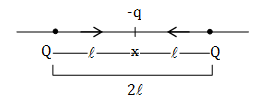$$F_{QQ}=\frac{1}{4\pi \varepsilon _{0}}.\frac{Q^{2}}{(2l)^{2}}$$
----------(1)

$$F_{qQ}=\frac{1}{4\pi \varepsilon _{0}}.\frac{qQ}{(l)^{2}}$$
----------------(2)

From eq. (1) and eq. (2)

Equilibrium condition eq.1 = 2

$$\frac{1}{4\pi \varepsilon _{0}}.\frac{Q^{2}}{4l^{2}}=\frac{1}{4\pi \varepsilon _{0}}.\frac{qQ}{(l)^{2}}$$

$$q=\frac{-Q}{4}$$

⇒ Therefore the correct answer is a

Question 7: The increase in electrostatic potential energy of a dipole of moment p when it is taken from parallel to anti-parallel orientation in an electric field E is

1. a.2pE
2. b.3pE
3. c.5pE
4. d. 6pE

Solution:

U=-pEcosθ

Dipole moment = p

Electric field = E

Now find the value of U1and U2

U1=-pEcos0 [cos 0o = 1]

U1=-pE x 1

U1=-pE

And

U2=-pEcos1800 [cos 0o = 1]

U2=-pE x(- 1)

U2=+pE

ΔU =(U2-U1)

ΔU = pE+pE =2pE

Increase in potential energy ΔU =2pE

Question 8: An n-p-n transistor of current gain 80 in common emitter mode gives emitter current equal to 8.1mA. The base current is

1. a.0.1μA
2. b.0.01mA
3. c.0.1mA
4. d.0.01μA

Solution:

$$\beta=\frac{I_{C}}{I_{B}}=80$$
…… (1)

IE =8.1 mA

For CE configuration

IC = 8.1 mA

$$I_{B}=\frac{I_{C}}{\beta}=\frac{8.1\times 10^{-3}A}{80}$$

IB = 0.1 mA

Therefore, the correct answer is (c)

Question 9: If a bar magnet of magnetic moment M is kept in a uniform magnetic field B, its time period of oscillation is T. In the same magnetic field, the time period of another magnet of same dimension and same mass but of moment M/4 is,

1. a. T
2. b. 2T
3. c. T/2
4. d. T/4

Solution: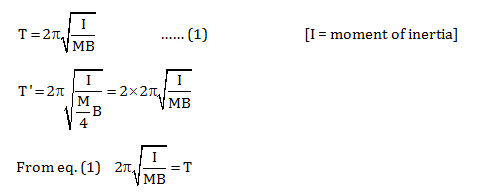Now

T’ = 2T

Therefore, the correct answer is (b)

Question 10: The magnetic field intensity at the centre of cubical cage of identical wires of length ‘a’ due to a current I flowing as shown in the figure is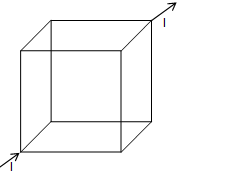1. a. 2I/a
2. b. √2I/a
3. c. 0
4. d. I/2a

Solution:

For symmetrical current distribution, magnetic field at the centre due to all current carrying conductors will get cancelled.

Hence Bnet = 0

Question 11: A micro-ammeter gives full scale deflection at 100μA. Its resistance is 2kΩ. The resistance required to convert it to a voltmeter reading 1V is

1. a. 1k Ω in series
2. b. 8k Ω in series
3. c. 1 k Ω in parallel
4. d. 8k Ω in parallel

Solution:

Since we know that to convert a Galvanometer into voltmeter a resistance is connected in series with it. (Galvanometer)

∵ V = IR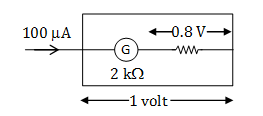I = 100μA (Given)

R = 2k Ω (Given)

∴ V = 100×10-6 ×2 ×103

V = 0.2 volt

Resultant voltage across the resistance which is connected in series with galvanometer (or micro ammeter) will be = 1 – 0.2 = 0.8 volt.

∴ Now resistance required to convert in voltmeter.

IxR = (0.8 V)

10-4x R= 0.8

R = (8 x 103) Ω in series

Question 12: An electron of mass ‘m’ is accelerated by a potential difference V and the corresponding de-Broglie wavelength is λ. The de Broglie wavelength of a proton of mass M if it is accelerated by the same potential difference is,

1. a. 0
2. b.
$$\lambda \left ( \frac{M}{m} \right )$$
3. c.
$$\lambda \left ( \frac{m}{M} \right )$$
4. d.
$$\lambda \sqrt{\frac{M}{m}}$$

Solution:

$$K.E.= \frac{1}{2}mv^{2}$$

$$\Rightarrow m\times \frac{1}{2}mv^{2}=v\times m$$

p2 = 2vm

$$p = \sqrt{2vm}$$

De-Broglie wave length formula

$$\lambda =\frac{h}{p}$$

$$\lambda =\frac{h}{\sqrt{2vm}}$$

Same potential difference

$$\lambda \propto \frac{h}{\sqrt{m}}$$

Now

$$\frac{\lambda }{\lambda _{p}}=\frac{\sqrt{M}}{\sqrt{m}}$$

$$\lambda _{p}=\lambda \frac{ \sqrt{m}}{\sqrt{M}}$$

Therefore the correct answer is (c)

Question 13: If I1 and I2 be the sizes of real images for two positions of a convex lens between object and screen, then the size of the object is

1. a. I1/I2
2. b. I1 I2
3. c.
$$\sqrt{l_{1}l_{2}}$$
4. d.
$$\sqrt{l_{1}/l_{2}}$$

Solution:

$$\frac{l_{1}}{O}=\frac{v}{u}$$
----------(1)

$$\frac{l_{2}}{O}=\frac{v}{u}$$
----------(2)

From eq. (1) and (2)

$$\Rightarrow \frac{l_{1}}{O}=\frac{O}{l_{2}}$$

$$\Rightarrow O^{2}=l_{1}l_{2}$$

$$\Rightarrow O=\sqrt{l_{1}l_{2}}$$

Therefore, the correct answer is (c)

Question 14: Impedance of a coil having inductance 0.4 H at frequency of 50 Hz will be

1. a. 20π Ω
2. b. 40π Ω
3. c. 2π Ω
4. d. 4π Ω

Solution:

$$Z=\sqrt{(X_{L}-X_{C})^{2}+R^{2}}$$
[Xc= 0, R =0]

Now

Z= XL = ωL

ωL =2 πF

Z = 2 x π x 50 x 0.4

Z = 40 π Ω

Therefore, the correct answer is (b)

Question 15: 1000 drops of water of radius 1 cm each carry a charge of 10 esu combine to form a single drop. The capacitance increases thereby

1. a. 1 time
2. b. 10 times
3. c. 100 times
4. d. 1000 times

Solution:

1 esu = 3.333 x 10-10 C

Volume of single big drop = vol. of 1000 small drops

$$\frac{4}{3}\pi R^{3}=\frac{4}{3}\pi r^{3}\times 1000$$

$$R^{3}={1000r^{3}}$$

R =10r

Electric potential on surface of drop

$$V_{s}=K\frac{q}{r}$$
(K= Coulomb’s constant)

Comparing with Q= C.V

$$C=\frac{q}{V_{s}}=\frac{q}{\frac{Kq}{r}}$$

C = r/K ----------(1)

Similarly capacitance of big drop

Cb = R/K …… (2)

From eq. (1) and (2)

$$\frac{C_{b}}{C}=\frac{R}{r}$$

$$\frac{C_{b}}{C}=\frac{10r}{r}$$

Cb =10 C

∴ Capacitance is 10 times

Therefore, the correct answer is (b)

Question 16: Light is an electromagnetic wave whose

1. a. E and B are parallel and both are perpendicular to the direction of propagation
2. b. E and B are mutually perpendicular and the direction of propagation is parallel to E
3. c. E and B and the direction of propagation are mutually perpendicular
4. d. E and B are mutually perpendicular and the direction of propagation is parallel to B

Solution:

As we know that the electromagnetic waves are the waves in which electric field E , magnetic field B and the direction of propagation of electromagnetic wave are mutually perpendicular light is electromagnetic waves so it will also contains these properties.

Question 17: In Bohr model of an atom, two electrons move round the nucleus in circular orbits of radii in the ratio 1: 4. The ratio of their kinetic energies are

1. a. 1 : 4
2. b. 4 : 1
3. c. 8 : 1
4. d. 1 : 8

Solution:

Since Kinetic Energy

$$E\propto \frac{1}{r}$$
where ,r = radius

E2r2 = E1r1

$$\frac{E_{1}}{E_{2}}=\frac{r_{2}}{r_{1}}\Rightarrow \frac{4}{1}$$

Therefore, the correct answer is (b)

Question 18: If a Young’s double slit experiment were conducted inside water instead of air, the fringe width would

1. a. increase
2. b. decrease
3. c. remains same
4. d. become zero

Solution:

In water, speed of light decreases

Since frequency remains same, therefore the wavelength decrease

The formula for fringe width is

$$\frac{\lambda D}{d}$$

Thus, fringe width decreases in water because wavelength of light decreases.

Therefore, the correct answer is (b)

Question 19: A photon of energy 8eV is incident on a metal plate with threshold frequency 1.6 × 1015 Hz. The maximum kinetic energy of the emitted photo electrons is (given h = 6 × 10 –34 Js)

1. a. 6 eV
2. b. 1.6eV
3. c. 1.2eV
4. d. 2 eV

Solution:

v0 = Threshold frequency

$$\phi =uv_{0}=\frac{6\times 10^{-34}\times 1.6\times 10^{15}}{1.6\times 10^{-19}}ev$$

= 6 x 10-34 x 1034

= 6ev

Kmax = E - φ

= 8ev - 6ev = 2ev

Therefore, the correct answer is (d)

Question 20: The ratio of the masses of two planets is 2:3 and the ratio of their radii is 3:2. The ratio of acceleration due to gravity on these two planets is

1. a. 9 : 4
2. b. 4 :9
3. c. 2 : 3
4. d. 3 : 2

Solution:

Acceleration due to gravity on the first planet

$$g_{1}=\frac{GM_{1}}{R_{1}^{2}}$$

Acceleration due to gravity on the second planet

$$g_{2}=\frac{GM_{2}}{R_{2}^{2}}$$

$$\frac{g_{1}}{g_{2}}=\frac{M_{1}}{M_{2}}\times \frac{R_{2}^{2}}{R_{1}^{2}}$$

$$\Rightarrow \frac{2}{3}\times \left [ \frac{2}{3} \right ]^{2}\Rightarrow \frac{8}{27}$$

Therefore, none of the options are correct

Question 21: A mass m rests on another mass M. The co-efficient of static friction between the surfaces of m and M is μ. M rests on a smooth frictionless horizontal plane. The maximum force applied horizontally on M for which m will move along with M without slipping is,

1. a. mg + μmg
2. b. (M + m) μg
3. c.
$$\frac{mM}{M+m}\mu g$$
4. d. μmg

Solution: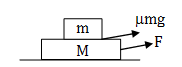F- μN1=(m+M)a

$$a=\frac{F-\mu_2 (m+M)g)}{(m+M)}$$

$$a=\frac{F}{(m+M)}-\mu_2 g$$
---------(1)

Maximum value of acceleration

ma =μ1mg

a = μ1g

Value of 'a' put in eq. (1)

$$\frac{F}{m+M}-\mu _{2}g=\mu _{1}g$$

$$F_{max}=(\mu _{1}g+\mu _{2}g).(m+M)$$
(Because, μ1 + μ2 =a)

Fmax = μg (m+M)

Therefore, the correct answer is (b)

Question 22: The dimension of co-efficient of viscosity η is

1. a. [MLT–2]
2. b. [M–1LT–1]
3. c. [M–1LT–2]
4. d. [ML–1T–1]

Solution:

Coefficient of viscosity (η) = fr/Av …… (1)

Where;

F = tangential force

r = distance between the layers,

v = velocity

Dimensional formula of :

Force = M1L1T-2

Area = M0L2T0

Distance = M0L1T0

Velocity = M0L1T-1

Put all these values in eq. (1)

We get,

$$(\eta )={\frac{[M^{1}L^{1}T^{-2}][M^{0}L^{1}T^{0}]}{[M^{0}L^{2}T^{0}][M^{0}L^{1}T^{-1}]}}$$

$$(\eta )=[M^{1}L^{-1}T^{-1}]$$

SI unit = Pascal second

Therefore, the correct answer is (d)

Question 23: An explosive of mass 9 kg is divided in two parts. One part of mass 3 kg moves with velocity of 16 m/s. The kinetic energy of other part will be

1. a. 192 J
2. b. 162 J
3. c. 150 J
4. d. 200 J

Solution:

Given mass of explosive = M = 9kg

Initial value of explosive = 0 m/s

Mass of smaller fragment = 3kg

$$\rightarrow$$
m1

Mass of bigger fragment = 6kg

$$\rightarrow$$
m2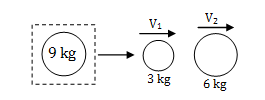3v1 =6v2

v2 =3v1/6

= (3 x16)/6 = 8m/s

$$(KE)6 Kg = \frac{1}{2}\times 6\times (8)^{2}$$

= 192 J

Therefore, the correct answer is (a)

Question 24: The length of a wire is l1 when tension is T1 and is l2 when tension is T2. The length of the wire without any tension is

1. a.
$$\frac{l_{2}T_{1}-l_{1}T_{2}}{T_{1}-T_{2}}$$
2. b.
$$\frac{l_{1}T_{1}-l_{2}T_{2}}{T_{1}-T_{2}}$$
3. c.
$$\frac{l_{1}T_{1}}{T_{2}-T_{1}}$$
4. d.
$$\frac{l_{2}T_{2}}{T_{1}-T_{2}}$$

Solution:

Change in length in the first case = (l1 -l)

Change in length in second case = (l2 - l)

Now, young modulus = Normal stress/ Longitudinal strain

$$\gamma =\frac{T/A}{\Delta l/l}$$

Where

γ= Young's Modulus

T = Tension

A = Area

ΔL = Change in length

l = Original length

Therefore,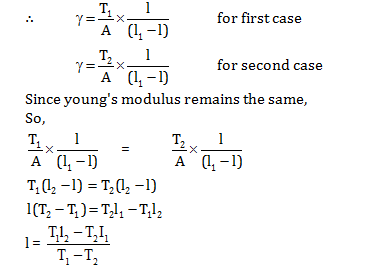Question 25: A particle of mass m is moving in a circular orbit of radius r in a force field given by

$$\vec{F}=-\frac{k}{r^{2}}\hat{r}$$
. The angular momentum L of the particle about the centre varies as

1. a.
$$L\propto \sqrt{r}$$
2. b.
$$L\propto r^{3/2}$$
3. c.
$$L\propto \frac{1}{\sqrt{r}}$$
4. d.
$$L\propto r^{1/3}$$

Solution:

mv2r2 =kr

$$\Rightarrow \frac{mv^{2}}{r}=\frac{k}{r^{2}}$$

$$\Rightarrow v=\sqrt{\frac{k}{mr}}$$

$$L=mvr =\sqrt{mrk}$$

Thus, we get

$$L\propto \sqrt{r}$$

Question 26: A sphere, a cube and a thin circular plate, all made of the same material and having the same mass, are initially heated to a temperature of 200°C. When left in air at room temperature, the following cools fastest.

1. a. Sphere
2. b. Cube
3. c. Plate
4. d. All of these

Solution: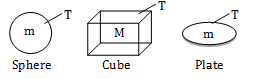$$\rho =\frac{mass}{volume}$$

$$\Rightarrow \rho$$
same for all objects

As volume and mass same for 3 objects. Thickness of plate

$$\rightarrow$$
Least
$$\rightarrow$$
surface area = Max.

According to Stefan’s loss heat law

$$H\propto AT^{4}$$

Hsphere = Hcube = Hplate = Asphere : Acube : Aplate

∴Plate will cool fast

Sphere cools slowest

Therefore, the correct answer is (c)

Question 27: Two sound waves of wavelengths 50 cm and 51 cm produce 12 beats per second. The velocity of sound is

1. a. 306ms–1
2. b. 331 ms–1
3. c. 340 ms–1
4. d. 360ms–1

Solution:

Wavelength of first wave λ1 = 0.5 m

Wavelength of second wave λ2 = 0.5 m

Frequency of beat = 12

We know that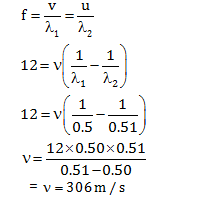Therefore, the correct answer is (a)

Question 28: Air is expanded from 50 litre to 150 litre at 2 atmospheric pressure (1 atm pressure = 105 kgm–2). The external work done is

1. a. 200 J
2. b. 2000 J
3. c. 2 × 104 J
4. d. 2 × 104 J

Solution:

v1 = 50 litre = 50 × 10-3

v2 = 150 litre = 150 ×10-3

W = P ΔV

⇒P (V2 – V1)

P = 2 atm = 2×105 N-2

W = 2×105 (150-50) ×10-3

W = 2×105 × 100 ×10-3

W = 2 × 104 J

Therefore, the correct answer is (c)

Question 29: The Young’s modulus of a metal is 2 × 1012 dyne/cm2 and its breaking stress is 11000 kg/cm2. In case of longitudinal strain the maximum energy that can be stored per cubic metre of this metal is approximately (Assume g = 10 m/s2)

1. a. 58.28 × 105 J
2. b. 30.25 × 105 J
3. c. 37.15 × 105 J
4. d. 15.15 × 105 J

Solution:

Energy density,

$$\frac{du}{dv}=\frac{(Stress)^{2}}{2y}$$

$$Stress =\frac{11000g}{10^{-4}}N/m^{2}$$

Now

$$\Rightarrow \frac{(\left [\frac{11000g}{10^{-4}}N/m^{2} \right ])^2}{2\times 2\times 10^{12}\times \frac{10^{-5}}{10^{-4}}}$$

$$Energy= \frac{121 \times 10^{6}\times 10^{2}}{4\times 10^{12}\times 10^{-1}\times 10^{-8}}$$

$$= \frac{30.25 \times 10^{8}}{ 10^{-8}\times 10^{12}\times 10^{1}}$$

$$= \frac{30.25 \times 10^{17}}{ 10^{12}}$$

Energy =30.25 x105 J

Therefore, the correct answer is (b)

Question 30: When a body moves in a circular orbit its total energy is

1. a. positive
2. b. negative
3. c. Zero
4. d. infinite

Solution:

Suppose an object with mass m doing a circular orbit around a much heavier object with mass M.

Now we know its potential energy, it's

$$U= \frac{-GMm}{R}$$
-----------(1)

As we know that the relation between linear velocity and angular velocity is given by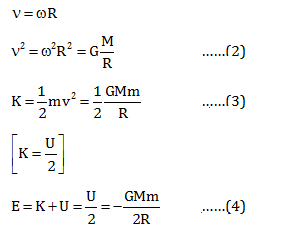So the total energy is always negative

Therefore, the correct answer is (b)

Question 31: Equivalent resistance of the given infinite circuit is

1. a. 4 Ω
2. b. 2 Ω
3. c. Infinity
4. d. Greater than 4 Ω but less than 6 Ω

Solution: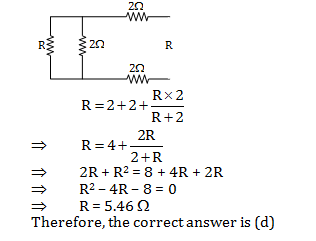Question 32: The ionization potential of hydrogen is –13.6 eV. The energy required to excite the electron from the first to the third orbit is approximately

1. a. 10.2 J
2. b. 12.09 × 10–6 J
3. c. 19.94 J
4. d. 19.34 × 10–19 J

Solution:

$$E=\frac{-13.6}{n^{2}}=\frac{-13.6}{9}=-1.5 eV$$

We know that energy required

ΔE = E2-E1

ΔE = 1.51 + 13.6 = 12.08 ev

So, ΔE = 12.08 eV

= 12.08 x 1.6 x 10-19 J

Therefore, the correct answer is (d)

Question 33: Assume that the Earth rotates in a circular orbit round the Sun in 365 days. If the mass of the sun gets doubled but the radius of the orbit remains unchanged, the length of the year would be approximately

1. a. 183 days
2. b. 258 days
3. c. 516 days
4. d. 730 days

Solution:

Since time period of revolution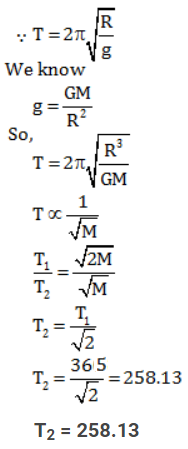Question 34: For the following set(s) of forces (in the same unit) the resultant can never be zero

1. a. 10,10,10
2. b. 10,10,20
3. c. 10,20,30
4. d. 10,20,40

Solution:

Option (a)

In 1st case, if 10N and 10N are at 120° then resultant of these two is 10N. Then the 3rd force if acted opposite to the resultant then net force is zero.

In 2nd case, if 10N and 10N are in same direction and 20N is opposite to both of them then resultant is zero.

In 3rd case, if 10N and 20N are acted at an angle of cos-1(-1/4) then the resultant is 20N. Then the third force if acted opposite to the resultant then net force is zero.

Explanation of third case: R2 = P2+Q2+2PQcosθ or (20)2 = (10)2+(20)2+2(10)(20)cosθ

So, cosθ = (400 - 500)/400 = -1/4.

This is possible value of cosθ as it lies in the interval [-1,1].

Any such situation is not possible in 4th case. Hence, the resultant cannot be zero in 4th case.

Question 35: A particle of mass ‘m’ and carrying a charge ‘q’ enters with a velocity ‘v’ perpendicular to a uniform magnetic field. The time period of rotation of the particle

1. a. Decreases with increase of velocity v
2. b. Increases with increase of radius of the orbit
3. c. Depends only on magnetic field
4. d. Depends on magnetic field and (q/m) of the particle

Solution:

$$T=\frac{2\pi m}{qB}$$

Depends on B and (q/m) of particle

Therefore, the correct answer is (d)

Question 36: At any instant t current i through a coil of self-inductance 2 mH is given by i = t2e–t. The induced e.m.f. will be zero at time

1. a. 1 sec
2. b. 2 sec
3. c. 3 sec
4. d. 4 sec

Solution: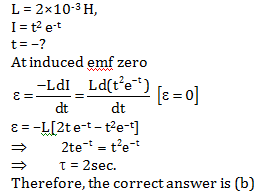Question 37: A and B are two parallel sided transparent slabs of refractive indices n1 and n2 respectively. A ray is incident at an angle θ on the surface of separation of A and B, and after refraction from B into air grazes the surface of B. Then

1. a. sin θ = 1/n2
2. b. sin θ = 1/n1
3. c. sin θ = n2/n1
4. d. sin θ = n1/n2

Solution:

$$n_{1}sin\theta =n_{2}sin\theta$$

Also,

$$sin\theta =\frac{1}{n_{2}}$$

$$\Rightarrow n_{1}sin\theta =\frac{n_{2}}{n_{2}}=1$$

$$\Rightarrow sin\theta =\frac{1}{n_{1}}$$

Question 38: The pair of parameters temperature T, pressure P, volume V and work W characterizes the thermodynamic state of matter

1. a. T, P
2. b. T, V
3. c. T, W
4. d. P, W

Solution:

We know that

The relation between the thermodynamics variable (P,V,T) of the system is called equation of state where in –

PV = nRT

(P,V,T) represent thermodynamic state of matter work does not represent thermodynamic state of matter.

Therefore, the correct answer is (a, b)

Question 39: Equation of a wave is given by y = 10–4 sin(60t + 2x), x and y in metre and t is in second. Then

1. a. Wave is propagating along the negative x direction with velocity 30 m/s
2. b. Wavelength is π metre
3. c. Frequency is 30/π Hz
4. d. Wave is propagating along positive x direction with velocity 60 m/s

Solution:

$$V=\frac{dy}{dt}=10\times 60\times cos(60t+2x)$$

Velocity = ω/K = 60/2 =30m/s

y is the form Asin(ωt+kx) , velocity is negative

K = 2,

$$\lambda =\frac{2\pi }{K}$$

$$\Rightarrow \lambda =\pi m$$

$$f=\frac{\omega }{2\pi }=\frac{60}{2\pi }=\frac{30}{\pi }Hz$$

Therefore, the correct answer is (a,b,c)

Question 40: An electric dipole is placed in a non-uniform electric field E. The electric field is along x direction. The dipole will experience

1. a. A torque when the dipole is parallel to E
2. b. A torque when the dipole makes an angle with E
3. c. A force perpendicular to E
4. d. A force when the dipole is parallel to E

Solution:

1. Solution: (b,d)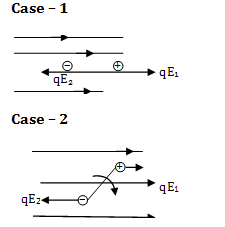In the question the electric field is in x-direction and a dipole is placed in this electric field.

Here we can consider 2 cases.

Case – 1 and Case – 2

In Case – 1

Dipole is placed parallel to the electric field so dipole will experience a net force and in this case the line of force is same. So there will be no torque for the dipole but as electric field is non uniform so there will be a net force on the dipole i.e.,

$$F=q(E_{1}-E_{2})\neq 0$$

In Case – 2

We placed the dipole in electric field (non-uniform) making an angle with electric field. So there will be definitely a torque working and that will not be equal to zero.

$$E_{1}=E_{2}\, or\, E_{1}\neq E_{2}$$

$$\tau \neq 0$$

### WBJEE 2016 Physics Question Paper with Solutions